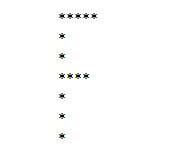# How to print alphabet latters In Php?

It is very easy to print alphabet latter in php. This is a type of matrix in which we can print rows and columns. You can make the alphabet latter using for loop and if condition in php. This can improve the logic of Fresher. Check below the programming syntax in front of output:

 ``` 1 && \$j < 4))) { echo "*"; } else { echo "  "; } } echo "
"; }?>```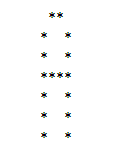``` 1)) || (\$j == 5 && (\$i != 0 && \$i != 3 && \$i != 6))) echo "*"; else echo "  "; } echo "
"; }?>```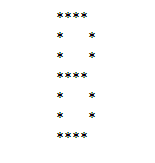``` 1 && \$j < 5)) || (\$j == 5 && (\$i == 1 || \$i == 5))) echo "*"; else echo "  "; } echo "
"; }?>```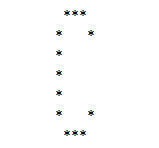``` 1 && \$j < 6)) || (\$i == 3 && \$j > 1 && \$j < 5)) echo "*"; else echo "  "; } echo "
"; }?>```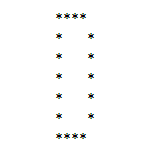``` 1 && \$j < 6)) || (\$i == 3 && \$j > 1 && \$j < 5)) echo "*"; else echo "  "; } echo "
"; }?>```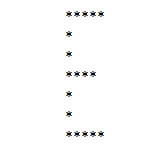``` 1 && \$j < 6)) || (\$i == 3 && \$j > 1 && \$j < 5)) echo "*"; else echo "  "; } echo "
"; }?>```